## Example Questions

← Previous 1

### Example Question #1 : Inequalities

|12x + 3y| < 15

What is the range of values for y, expressed in terms of x?

5 – 4x < y < 5 + 4x

y > 15 – 12x

5 + 4x < y < 5 – 4x

–5 – 4x < y < 5 – 4x

y < 5 – 4x

–5 – 4x < y < 5 – 4x

Explanation:

Recall that with absolute values and "less than" inequalities, we have to hold the following:

12x + 3y < 15

AND

12x + 3y > –15

Otherwise written, this is:

–15 < 12x + 3y < 15

In this form, we can solve for y. First, we have to subtract x from all 3 parts of the inequality:

–15 – 12x < 3y < 15 – 12x

Now, we have to divide each element by 3:

(–15 – 12x)/3 < y < (15 – 12x)/3

This simplifies to:

–5 – 4x < y < 5 – 4x

### Example Question #1 : How To Find The Solution To An Inequality With Subtraction

|4x + 14| > 30

What is a possible valid value of x?

4

7

–11

1

–3

7

Explanation:

This inequality could be rewritten as:

4x + 14 > 30  OR 4x + 14 < –30

Solve each for x:

4x + 14 > 30; 4x > 16; x > 4

4x + 14 < –30; 4x < –44; x < –11

Therefore, anything between –11 and 4 (inclusive) will not work. Hence, the answer is 7.

### Example Question #3 : Inequalities

Given the inequality,  |2x – 2|  >  20,

what is a possible value for x?

–8

10

0

11

–10

–10

Explanation:

For this problem, we must take into account the absolute value.

First, we solve for 2x – 2 > 20.  But we must also solve for 2x – 2 < –20 (please notice that we negate 20 and we also flip the inequality sign).

First step:

2x – 2 > 20

2x > 22

x > 11

Second step:

2x – 2 < –20

2x < –18

x < –9

Therefore, x > 11 and x < –9.

A possible value for x would be –10 since that is less than –9.

Note: the value 11 would not be a possible value for x because the inequality sign given does not include an equal sign.

### Example Question #4 : Inequalities

Solve for.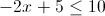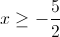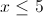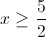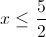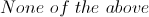Explanation:

Move +5 using subtraction rule which will give you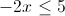Divide both sides by 2 (using division rule) and you will get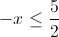which is the same as### Example Question #5 : Inequalities

If 2 more thanis a negative integer and if 5 more thanis a positive integer, which of the following could be the value of?-7Explanation: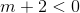and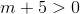, so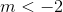and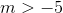.  The only integers betweenandareand.

### Example Question #2 : Inequalities

If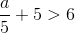, which of the following MUST be true?

I.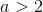II.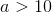III.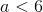I, II, and III

III only

II only

I only

I and II only

I only

Explanation:

Subtract 5 from both sides of the inequality: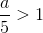Multiply both sides by 5:Therefore only I must be true.

### Example Question #7 : Inequalities

Which of the following is equivalent to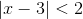?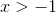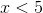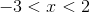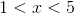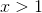Explanation:

Solve for both x – 3 < 2 and –(x – 3) < 2.

x – 3 < 2 and –x + 3 < 2

x < 2 + 3 and –x < 2 – 3

x < 5 and –x < –1

x < 5 and x > 1

The results are x < 5 and x > 1.

Combine the two inequalities to get 1 < x < 5

### Example Question #8 : Inequalities

Given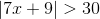, what is a possible value of?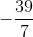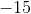Explanation:

In order to find the range of possible values for, we must first consider that the absolute value applied to this inequality results in two separate equations, both of which we must solve: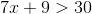and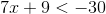.

Starting with the first inequality: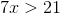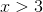Then, our second inequality tells us that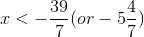Therefore,is the correct answer, as it is the only value above for which(NOT greater than or equal to) or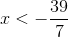(NOT less than or equal to).

### Example Question #1 : Inequalities

The cost, in cents, of manufacturingpencils is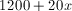, where 1200 is the number of cents required to run the factory regardless of the number of pencils made, and 20 represents the per-unit cost, in cents, of making each pencil. The pencils sell for 50 cents each. What number of pencils would need to be sold so that the revenue received is at least equal to the manufacturing cost?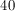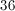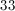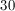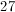Explanation:

If each pencil sells at 50 cents,pencils will sell at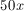. The smallest value ofsuch that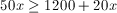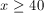### Example Question #9 : Inequalities

Solve for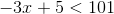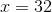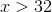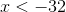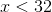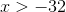The correct method to solve this problem is to substract 5 from both sides. This gives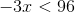.
This gives the answer.